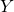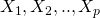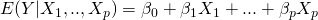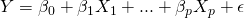# Assumptions for linear regression

Linear regression is one of the most commonly used statistical methods; it allows us to model how an outcome variabledepends on one or more predictor (sometimes called independent variables). In particular, we model how the mean, or expectation, of the outcomevaries as a function of the predictors:Equivalently, the linear model can be expressed by:wheredenotes a mean zero error, or residual term. To carry out statistical inference, additional assumptions such as normality are typically made.

However, a common misconception about linear regression is that it assumes that the outcomeis normally distributed. Actually, linear regression assumes normality for the residual errors, which represent variation inwhich is not explained by the predictors. It may be the case that marginally (i.e. ignoring any predictors)is not normal, but after removing the effects of the predictors, the remaining variability, which is precisely what the residuals represent, are normal, or are more approximately normal.

So, inferential procedures for linear regression are typically based on a normality assumption for the residuals. However, a second perhaps less widely known fact amongst analysts is that, as sample sizes increase, the normality assumption for the residuals is not needed. More precisely, if we consider repeated sampling from our population, for large sample sizes, the distribution (across repeated samples) of the ordinary least squares estimates of the regression coefficients follow a normal distribution. As a consequence, for moderate to large sample sizes, non-normality of residuals should not adversely affect the usual inferential procedures. This result is a consequence of an extremely important result in statistics, known as the central limit theorem.

A further assumption made by linear regression is that the residuals have constant variance. That is, their variance does not change across different levels of the predictors. In contrast to the normality assumption, if the residuals do not satisfy the constant variance assumption, standard errors and confidence intervals (based on standard formulae) will be adversely affected, irrespective of whether the sample size is large or not. However even in this case, the ordinary least squares estimators are unbiased. If the constant variance assumption is violated, we should use the robust sandwich variance estimator.

### 4 thoughts on “Assumptions for linear regression”

1.On the assumption of normality of errors doesn’t imply normality of response: If we assume that the predictors are fixed i.e. non-random then surely the model can be written as:

Yi = E[ Yi |x ] + ei

where e~N(0,sigma2).

E[Yi|x] = Mui -> fixed (despite being conditional mean)-> non-random

Yi = Mui + ei -> This implies Yi is distributed as normal, specifically –> Y~N(Mui, sigma2)

•Thanks! I agree. In this post I was implicitly taking the covariates to be random rather than fixed. So when I was talking about the distribution of Y, I was meaning marginally with respect to the covariates.

2.What do you define as large enough sample size? I’ve heard n=50, 100, and 500 suggested as possible cut-offs.

Additionally, this is an amazing systematic review on misconceptions about the assumptions of linear regression. Of the 900 papers examined, the authors found that ~95% of them either incorrectly assessed for normality (assessed normality of DV but not residuals) or didn’t report assessing for violations at all:

https://www.ncbi.nlm.nih.gov/pmc/articles/PMC5436580/

This is a big issue in clinical research!

•